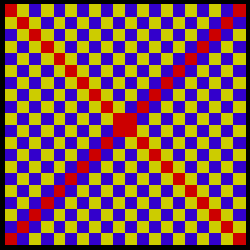### Color AssimilationThis figure is from Dr. Irtel .

Take two pieces of paper and place their edges as close as possible to both sides of one red diagonal. Note that the red square in the middle is the same as all the other squares on that diagonal. Now move your pieces of paper to expose mainly the red squares on the other diagonal. On this diagonal  all the red squares, including the center one, are again the same color red. Because the center square is common to both diagonals this exercise proves that all the red squares in both diagonals are exactly the same red color. Yet, when the pattern is seen as a whole, the two red diagonals appear different from each other.